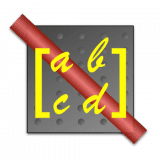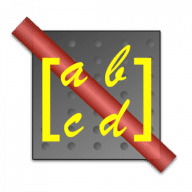ABCD
4.1

0.0# ABCD4.1

13 June 2013

Calculate properties of a laser beam propagating through optical elements.

## Overview

ABCD Gaussian Beam Propagation is an application that calculates the various properties of a laser beam propagating through simple optical elements. It displays the beam and the optical setup in a graphical window that can be manipulated by the user. A table shows the values at all points, they can be copied and used in plotting applications.

Features:

• User-defined inputs (wavelength, radius of curvature, size) and M-square (i.e. multimode equivalence)
• Calculate propagation through free space, dielectrics, lenses and mirrors. Use toolbars to add elements
• Calculate eigenmodes of a laser resonator
• Obtain the beam size, radius of curvature, waist size, waist position, and Rayleigh range at any point
• Copy and paste values (radius, beam size) into your own plotting program
• Copy the physical appearance of the setup as a PDF
• All calculations are performed using the ray matrix formalism (i.e. ABCD matrices) and therefore make the paraxial approximation

## What's new in ABCD

Version 4.1:
• Now works on OS X 10.6.x and 10.7.x
• Printing, in the form of a detailed calculation summary
• New optical elements: gaussian ducts
• Significant performance improvements, especially for large setups with many elements
• User can choose calculations performed before or after optical elements
• When drag-positioning elements, if multiple elements are selected, they move together
• Preferences for laser color, default property values, number of sampling points for graphs
• Slightly modified file format, automatically converted from prior version

#### Join over 500,000 subscribers.

Subscribe for our newsletter with best Mac offers from MacUpdate.

How would you rate ABCD app?